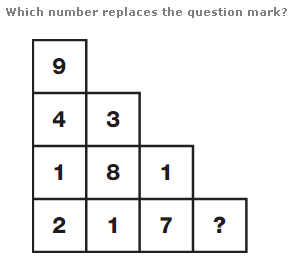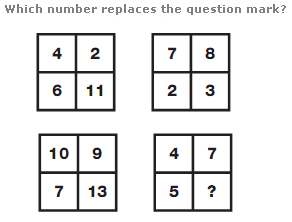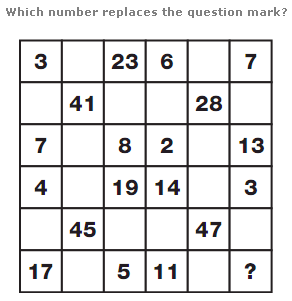# Puzzles - Number puzzles

Exercise : Number puzzles
244.:
4
Explanation
:
The average of the numbers in each column is always 4.

245.:
9
Explanation
:
Using the top two grids as a source, numbers in the lower left grid equal the sums of numbers in corresponding positions of the top two grids, subtracting 1 each time, and numbers in the lower right grid equal the differences between numbers in corresponding positions of the top two grids, adding 1 each time.

246.